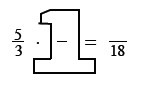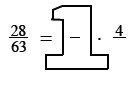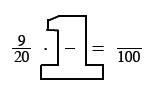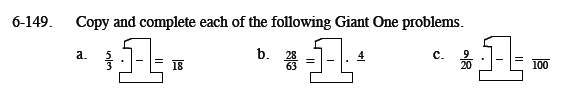### Home > MC1 > Chapter 6 > Lesson 6.3.3 > Problem6-149

6-149.
1. . Copy and complete each of the following Giant One problems. Homework Help ✎

1.2.3.By what number do you multiply three to get 18? This will be both the numerator and denominator in the Giant One, since the fraction is equal to one.

Follow the same method as in part (a). What number times 4 is equal to 28?

This problem is very similar to part (a). Remember that the Giant One is a fraction equal to one.